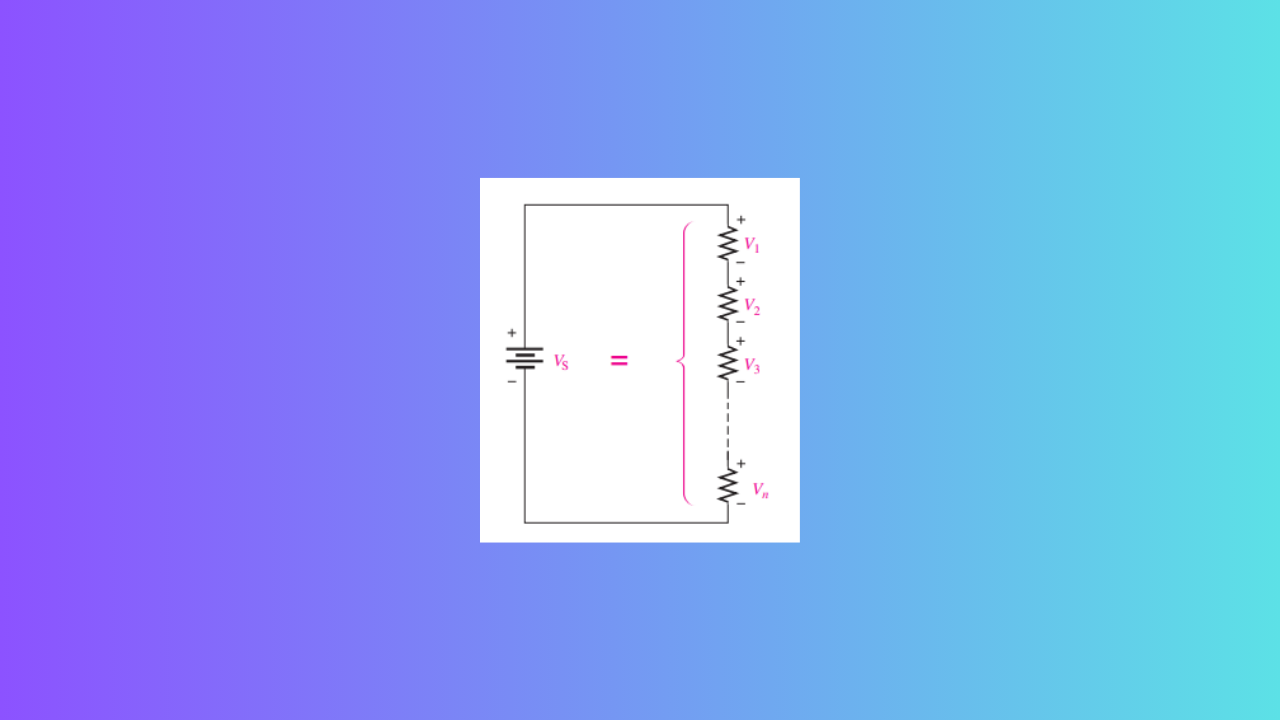## Key Principles of Series Circuits### Total Series Resistance

• The total series resistance is the sum of all resistors in the series circuit.
• The total resistance between any two points in a series circuit is equal to the sum of all resistors connected in series between those two points.
• If all resistors are of equal value, the total resistance equals the number of resistors multiplied by the resistance value of one resistor.

### Current in Series Circuit

• The current is the same at all points in a series circuit.

### Voltage Sources in Series

• Voltage sources in series add algebraically.

### Kirchhoff’s Voltage Law

• The sum of the voltage drops in a series circuit equals the total source voltage.
• The algebraic sum of all voltages around a closed single path is zero.

### Voltage Polarity

• The voltage drops in a circuit are always opposite in polarity to the total source voltage.

### Current Direction

• Current is defined to be out of the negative side of a source and into the positive side.
• Current is defined to be into the negative side of each resistor and out of the positive side.

### Voltage Divider

• A voltage divider is a series arrangement of resistors connected to a voltage source.
• The voltage drop across any resistor in the series circuit is divided down from the total voltage by an amount proportional to that resistance value in relation to the total resistance.
• A potentiometer can be used as an adjustable voltage divider.

### Power in Resistive Circuit

• The total power in a resistive circuit is the sum of all the individual powers of the resistors making up the series circuit.

### Voltage Referencing

• Voltages given with a single-lettered subscript are referenced to ground.
• When two different letters are used in the subscript, the voltage is the difference in the two points.

### Grounding

• Ground (common) is zero volts with respect to all points referenced to it in the circuit.
• Negative ground is used when the negative side of the source is grounded.
• Positive ground is used when the positive side of the source is grounded.

### Voltage Across Components

• The voltage across an open component always equals the source voltage.
• The voltage across a shorted component is always 0.
Share.
error: Content is protected !!